## Representation and Visualization of Data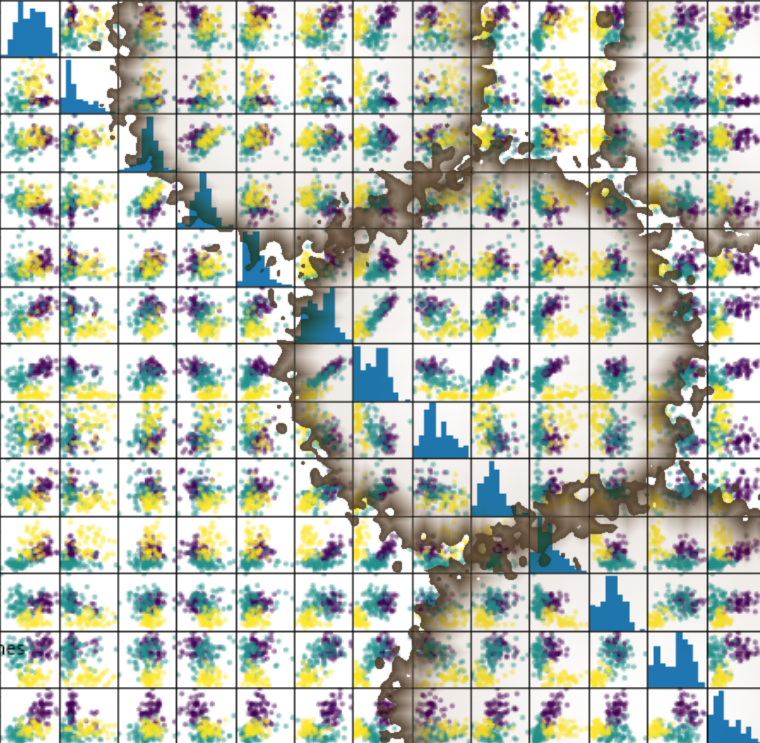Machine learning is about adapting models to data. For this reason we begin by showing how data can be represented in order to be understood by the computer.

At the beginning of this chapter we quoted Tom Mitchell's definition of machine learning: "Well posed Learning Problem: A computer program is said to learn from experience E with respect to some task T and some performance measure P, if its performance on T, as measured by P, improves with experience E." Data is the "raw material" for machine learning. It learns from data. In Mitchell's definition, "data" is hidden behind the terms "experience E" and "performance measure P". As mentioned earlier, we need labeled data to learn and test our algorithm.

However, it is recommended that you familiarize yourself with your data before you begin training your classifier.

Numpy offers ideal data structures to represent your data and Matplotlib offers great possibilities for visualizing your data.

In the following, we want to show how to do this using the data in the sklearn module.

### Iris Dataset, "Hello World" of Machine Learning

What was the first program you saw? I bet it might have been a program giving out "Hello World" in some programming language. Most likely I'm right. Almost every introductory book or tutorial on programming starts with such a program. It's a tradition that goes back to the 1968 book "The C Programming Language" by Brian Kernighan and Dennis Ritchie!

The likelihood that the first dataset you will see in an introductory tutorial on machine learning will be the "Iris dataset" is similarly high. The Iris dataset contains the measurements of 150 iris flowers from 3 different species:

• Iris-Setosa,
• Iris-Versicolor, and
• Iris-Virginica.

Iris SetosaIris VersicolorIris VirginicaThe iris dataset is often used for its simplicity. This dataset is contained in scikit-learn, but before we have a deeper look into the Iris dataset we will look at the other datasets available in scikit-learn.

## Loading the Iris Data with Scikit-learn

For example, scikit-learn has a very straightforward set of data on these iris species. The data consist of the following:

• Features in the Iris dataset:

1. sepal length in cm
2. sepal width in cm
3. petal length in cm
4. petal width in cm
• Target classes to predict:

1. Iris Setosa
2. Iris Versicolour
3. Iris Virginica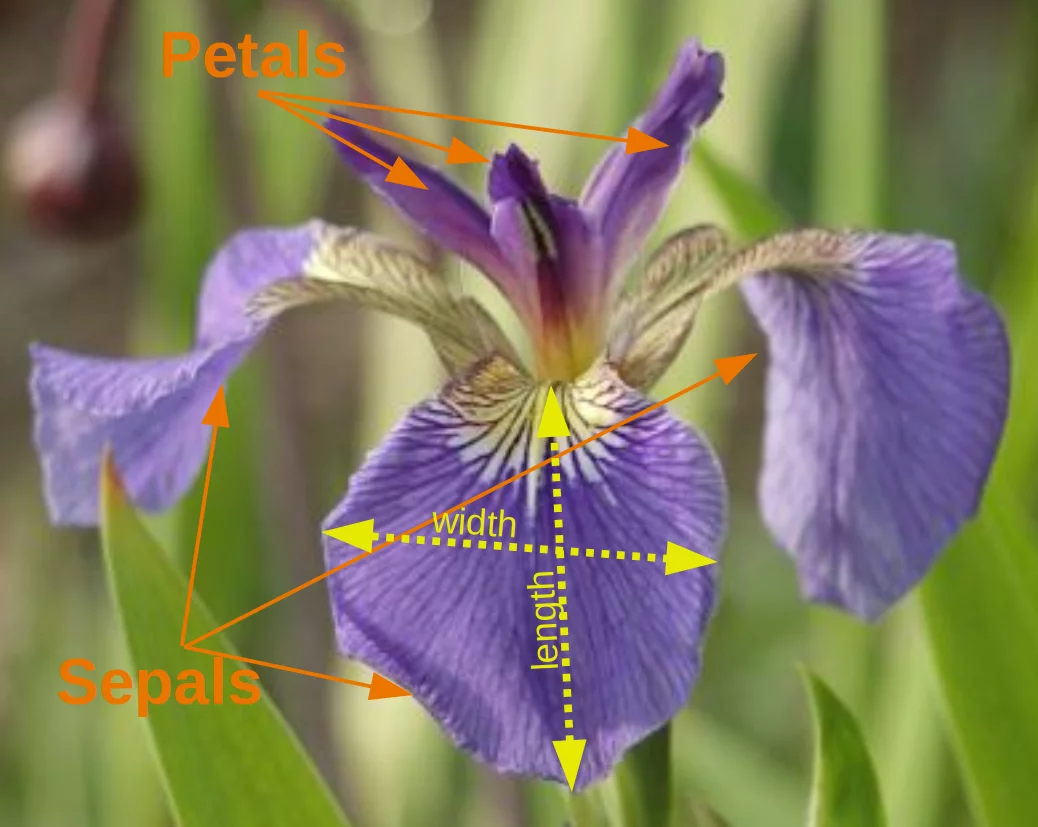scikit-learn embeds a copy of the iris CSV file along with a helper function to load it into numpy arrays:

from sklearn.datasets import load_iris
iris = load_iris()


The resulting dataset is a Bunch object:

type(iris)

Output:
sklearn.utils.Bunch

You can see what's available for this data type by using the method keys():

iris.keys()

Output:
dict_keys(['data', 'target', 'target_names', 'DESCR', 'feature_names', 'filename'])

A Bunch object is similar to a dicitionary, but it additionally allows accessing the keys in an attribute style:

print(iris["target_names"])
print(iris.target_names)

['setosa' 'versicolor' 'virginica']
['setosa' 'versicolor' 'virginica']


The features of each sample flower are stored in the data attribute of the dataset:

n_samples, n_features = iris.data.shape
print('Number of samples:', n_samples)
print('Number of features:', n_features)
# the sepal length, sepal width, petal length and petal width of the first sample (first flower)
print(iris.data)

Number of samples: 150
Number of features: 4
[5.1 3.5 1.4 0.2]


The feautures of each flower are stored in the data attribute of the data set. Let's take a look at some of the samples:

# Flowers with the indices 12, 26, 89, and 114
iris.data[[12, 26, 89, 114]]

Output:
array([[4.8, 3. , 1.4, 0.1],
[5. , 3.4, 1.6, 0.4],
[5.5, 2.5, 4. , 1.3],
[5.8, 2.8, 5.1, 2.4]])

The information about the class of each sample, i.e. the labels, is stored in the "target" attribute of the data set:

print(iris.data.shape)
print(iris.target.shape)

(150, 4)
(150,)

print(iris.target)

[0 0 0 0 0 0 0 0 0 0 0 0 0 0 0 0 0 0 0 0 0 0 0 0 0 0 0 0 0 0 0 0 0 0 0 0 0
0 0 0 0 0 0 0 0 0 0 0 0 0 1 1 1 1 1 1 1 1 1 1 1 1 1 1 1 1 1 1 1 1 1 1 1 1
1 1 1 1 1 1 1 1 1 1 1 1 1 1 1 1 1 1 1 1 1 1 1 1 1 1 2 2 2 2 2 2 2 2 2 2 2
2 2 2 2 2 2 2 2 2 2 2 2 2 2 2 2 2 2 2 2 2 2 2 2 2 2 2 2 2 2 2 2 2 2 2 2 2
2 2]

import numpy as np

np.bincount(iris.target)

Output:
array([50, 50, 50])

Using NumPy's bincount function (above) we can see that the classes in this dataset are evenly distributed - there are 50 flowers of each species, with

• class 0: Iris Setosa
• class 1: Iris Versicolor
• class 2: Iris Virginica

These class names are stored in the last attribute, namely target_names:

print(iris.target_names)

['setosa' 'versicolor' 'virginica']


### Data in sklearn (scikit-learn)

Data in scikit-learn is in most cases saved as two-dimensional Numpy arrays with the shape (n, m). Many algorithms also accept scipy.sparse matrices of the same shape.

• n: (n_samples) The number of samples: each sample is an item to process (e.g. classify). A sample can be a document, a picture, a sound, a video, an astronomical object, a row in database or CSV file, or whatever you can describe with a fixed set of quantitative traits.
• m: (n_features) The number of features or distinct traits that can be used to describe each item in a quantitative manner. Features are generally real-valued, but may be Boolean or discrete-valued in some cases.

The information about the class of each sample of our Iris dataset is stored in the target attribute of the dataset:

print(iris.target)

[0 0 0 0 0 0 0 0 0 0 0 0 0 0 0 0 0 0 0 0 0 0 0 0 0 0 0 0 0 0 0 0 0 0 0 0 0
0 0 0 0 0 0 0 0 0 0 0 0 0 1 1 1 1 1 1 1 1 1 1 1 1 1 1 1 1 1 1 1 1 1 1 1 1
1 1 1 1 1 1 1 1 1 1 1 1 1 1 1 1 1 1 1 1 1 1 1 1 1 1 2 2 2 2 2 2 2 2 2 2 2
2 2 2 2 2 2 2 2 2 2 2 2 2 2 2 2 2 2 2 2 2 2 2 2 2 2 2 2 2 2 2 2 2 2 2 2 2
2 2]


Beside of the shape of the data, we can also check the shape of the labels, i.e. the target.shape:

Each flower sample is one row in the data array, and the columns (features) represent the flower measurements in centimeters. For instance, we can represent this Iris dataset, consisting of 150 samples and 4 features, a 2-dimensional array or matrix $\mathbb{R}^{150 \times 4}$ in the following format:

$$\mathbf{X} = \begin{bmatrix} x_{1}^{(1)} & x_{2}^{(1)} & x_{3}^{(1)} & x_{4}^{(1)} \\ x_{1}^{(2)} & x_{2}^{(2)} & x_{3}^{(2)} & x_{4}^{(2)} \\ \vdots & \vdots & \vdots & \vdots \\ x_{1}^{(150)} & x_{2}^{(150)} & x_{3}^{(150)} & x_{4}^{(150)} \end{bmatrix}.$$

The superscript denotes the ith row, and the subscript denotes the jth feature, respectively.

Generally, we have $n$ rows and $k$ columns:

$$\mathbf{X} = \begin{bmatrix} x_{1}^{(1)} & x_{2}^{(1)} & x_{3}^{(1)} & \dots & x_{k}^{(1)} \\ x_{1}^{(2)} & x_{2}^{(2)} & x_{3}^{(2)} & \dots & x_{k}^{(2)} \\ \vdots & \vdots & \vdots & \vdots & \vdots \\ x_{1}^{(n)} & x_{2}^{(n)} & x_{3}^{(n)} & \dots & x_{k}^{(n)} \end{bmatrix}.$$
print(iris.data.shape)
print(iris.target.shape)

(150, 4)
(150,)


bincount of NumPy counts the number of occurrences of each value in an array of non-negative integers. We can use this to check the distribution of the classes in the dataset:

import numpy as np

np.bincount(iris.target)

Output:
array([50, 50, 50])

We can see that the classes are distributed uniformly - there are 50 flowers from each species, i.e.

• class 0: Iris-Setosa
• class 1: Iris-Versicolor
• class 2: Iris-Virginica

These class names are stored in the last attribute, namely target_names:

print(iris.target_names)

['setosa' 'versicolor' 'virginica']


## Visualising the Features of the Iris Data Set

The feauture data is four dimensional, but we can visualize one or two of the dimensions at a time using a simple histogram or scatter-plot.

from sklearn.datasets import load_iris
iris = load_iris()
print(iris.data[iris.target==1][:5])

print(iris.data[iris.target==1, 0][:5])

[[7.  3.2 4.7 1.4]
[6.4 3.2 4.5 1.5]
[6.9 3.1 4.9 1.5]
[5.5 2.3 4.  1.3]
[6.5 2.8 4.6 1.5]]
[7.  6.4 6.9 5.5 6.5]


### Histograms of the features

import matplotlib.pyplot as plt

fig, ax = plt.subplots()
x_index = 3
colors = ['blue', 'red', 'green']

for label, color in zip(range(len(iris.target_names)), colors):
ax.hist(iris.data[iris.target==label, x_index],
label=iris.target_names[label],
color=color)

ax.set_xlabel(iris.feature_names[x_index])
ax.legend(loc='upper right')
fig.show()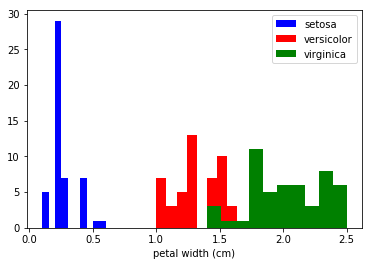### Exercise

Look at the histograms of the other features, i.e. petal length, sepal widt and sepal length.

### Scatterplot with two Features

The appearance diagram shows two features in one diagram:

import matplotlib.pyplot as plt
fig, ax = plt.subplots()

x_index = 3
y_index = 0

colors = ['blue', 'red', 'green']

for label, color in zip(range(len(iris.target_names)), colors):
ax.scatter(iris.data[iris.target==label, x_index],
iris.data[iris.target==label, y_index],
label=iris.target_names[label],
c=color)

ax.set_xlabel(iris.feature_names[x_index])
ax.set_ylabel(iris.feature_names[y_index])
ax.legend(loc='upper left')
plt.show()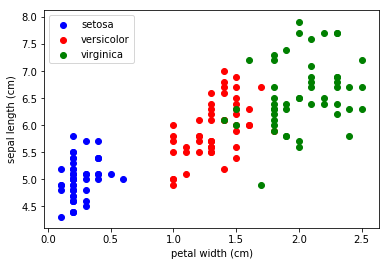### Exercise

Change x_index and y_index in the above script

Change x_index and y_index in the above script and find a combination of two parameters which maximally separate the three classes.

### Generalization

We will now look at all feature combinations in one combined diagram:

import matplotlib.pyplot as plt

n = len(iris.feature_names)
fig, ax = plt.subplots(n, n, figsize=(16, 16))

colors = ['blue', 'red', 'green']

for x in range(n):
for y in range(n):
xname = iris.feature_names[x]
yname = iris.feature_names[y]
for color_ind in range(len(iris.target_names)):
ax[x, y].scatter(iris.data[iris.target==color_ind, x],
iris.data[iris.target==color_ind, y],
label=iris.target_names[color_ind],
c=colors[color_ind])

ax[x, y].set_xlabel(xname)
ax[x, y].set_ylabel(yname)
ax[x, y].legend(loc='upper left')

plt.show()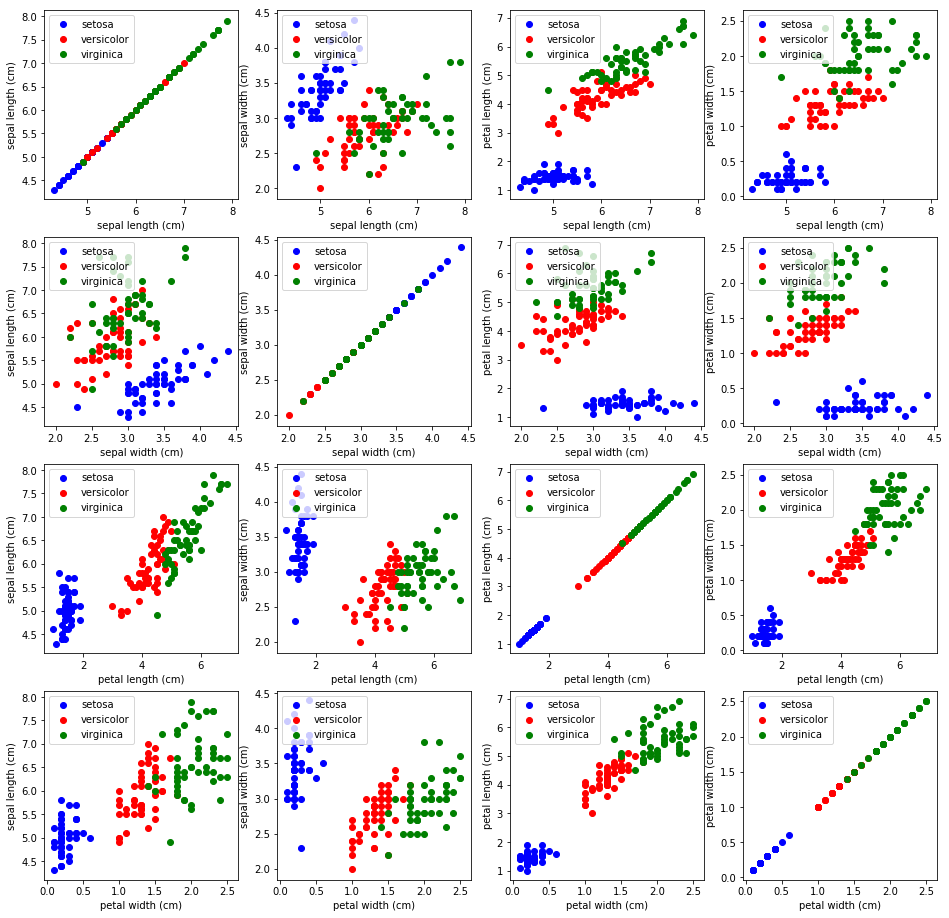## Scatterplot 'Matrices

Instead of doing it manually we can also use the scatterplot matrix provided by the pandas module.

Scatterplot matrices show scatter plots between all features in the data set, as well as histograms to show the distribution of each feature.

import pandas as pd

iris_df = pd.DataFrame(iris.data, columns=iris.feature_names)
pd.plotting.scatter_matrix(iris_df,
c=iris.target,
figsize=(8, 8)
);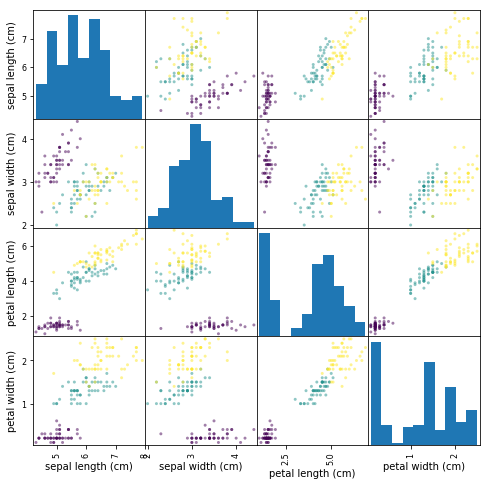### 3-Dimensional Visualization

import matplotlib.pyplot as plt
from sklearn.datasets import load_iris
from mpl_toolkits.mplot3d import Axes3D
iris = load_iris()
X = []
for iclass in range(3):
X.append([[], [], []])
for i in range(len(iris.data)):
if iris.target[i] == iclass:
X[iclass].append(iris.data[i])
X[iclass].append(iris.data[i])
X[iclass].append(sum(iris.data[i][2:]))

colours = ("r", "g", "y")
fig = plt.figure()
ax = fig.add_subplot(111, projection='3d')

for iclass in range(3):
ax.scatter(X[iclass], X[iclass], X[iclass], c=colours[iclass])
plt.show()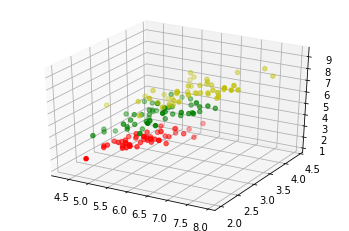## Other Available Data

Scikit-learn makes available a host of datasets for testing learning algorithms. They come in three flavors:

• Packaged Data: these small datasets are packaged with the scikit-learn installation, and can be downloaded using the tools in sklearn.datasets.load_*
• Downloadable Data: these larger datasets are available for download, and scikit-learn includes tools which streamline this process. These tools can be found in sklearn.datasets.fetch_*
• Generated Data: there are several datasets which are generated from models based on a random seed. These are available in the sklearn.datasets.make_*

You can explore the available dataset loaders, fetchers, and generators using IPython's tab-completion functionality. After importing the datasets submodule from sklearn, type

datasets.load_<TAB>



or

datasets.fetch_<TAB>



or

datasets.make_<TAB>



to see a list of available functions.

from sklearn import datasets


Be warned: many of these datasets are quite large, and can take a long time to download!

## Loading Digits Data

We will have a closer look at one of these datasets. We look at the digits data set. We will load it first:

from sklearn.datasets import load_digits
digits = load_digits()


Again, we can get an overview of the available attributes by looking at the "keys":

digits.keys()

Output:
dict_keys(['data', 'target', 'target_names', 'images', 'DESCR'])

Let's have a look at the number of items and features:

n_samples, n_features = digits.data.shape
print((n_samples, n_features))

(1797, 64)

print(digits.data)
print(digits.target)

[ 0.  0.  5. 13.  9.  1.  0.  0.  0.  0. 13. 15. 10. 15.  5.  0.  0.  3.
15.  2.  0. 11.  8.  0.  0.  4. 12.  0.  0.  8.  8.  0.  0.  5.  8.  0.
0.  9.  8.  0.  0.  4. 11.  0.  1. 12.  7.  0.  0.  2. 14.  5. 10. 12.
0.  0.  0.  0.  6. 13. 10.  0.  0.  0.]
[0 1 2 ... 8 9 8]


The data is also available at digits.images. This is the raw data of the images in the form of 8 lines and 8 columns.

With "data" an image corresponds to a one-dimensional Numpy array with the length 64, and "images" representation contains 2-dimensional numpy arrays with the shape (8, 8)

print("Shape of an item: ", digits.data.shape)
print("Data type of an item: ", type(digits.data))
print("Shape of an item: ", digits.images.shape)
print("Data tpye of an item: ", type(digits.images))

Shape of an item:  (64,)
Data type of an item:  <class 'numpy.ndarray'>
Shape of an item:  (8, 8)
Data tpye of an item:  <class 'numpy.ndarray'>


Let's visualize the data. It's little bit more involved than the simple scatter-plot we used above, but we can do it rather quickly.

# set up the figure
fig = plt.figure(figsize=(6, 6))  # figure size in inches
fig.subplots_adjust(left=0, right=1, bottom=0, top=1, hspace=0.05, wspace=0.05)

# plot the digits: each image is 8x8 pixels
for i in range(64):
ax = fig.add_subplot(8, 8, i + 1, xticks=[], yticks=[])
ax.imshow(digits.images[i], cmap=plt.cm.binary, interpolation='nearest')

# label the image with the target value
ax.text(0, 7, str(digits.target[i]))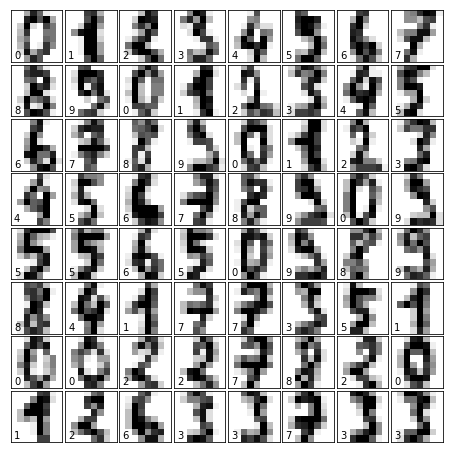### Exercises

#### Exercise 1

sklearn contains a "wine data set".

• Find and load this data set
• Can you find a description?
• What are the names of the classes?
• What are the features?
• Where is the data and the labeled data?

#### Exercise 2:

Create a scatter plot of the features ash and color_intensity of the wine data set.

#### Exercise 3:

Create a scatter matrix of the features of the wine dataset.

#### Exercise 4:

Fetch the Olivetti faces dataset and visualize the faces.

### Solutions

#### Solution to Exercise 1

Loading the "wine data set":

from sklearn import datasets

wine = datasets.load_wine()


The description can be accessed via "DESCR":

In [ ]:
print(wine.DESCR)


The names of the classes and the features can be retrieved like this:

print(wine.target_names)
print(wine.feature_names)

['class_0' 'class_1' 'class_2']
['alcohol', 'malic_acid', 'ash', 'alcalinity_of_ash', 'magnesium', 'total_phenols', 'flavanoids', 'nonflavanoid_phenols', 'proanthocyanins', 'color_intensity', 'hue', 'od280/od315_of_diluted_wines', 'proline']

data = wine.data
labelled_data = wine.target


#### Solution to Exercise 2:

from sklearn import datasets
import matplotlib.pyplot as plt

wine = datasets.load_wine()

features = 'ash', 'color_intensity'
features_index = [wine.feature_names.index(features),
wine.feature_names.index(features)]

colors = ['blue', 'red', 'green']

for label, color in zip(range(len(wine.target_names)), colors):
plt.scatter(wine.data[wine.target==label, features_index],
wine.data[wine.target==label, features_index],
label=wine.target_names[label],
c=color)

plt.xlabel(features)
plt.ylabel(features)
plt.legend(loc='upper left')
plt.show()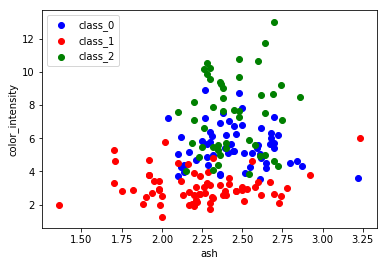#### Solution to Exercise 3:

import pandas as pd
from sklearn import datasets

wine = datasets.load_wine()
def rotate_labels(df, axes):
""" changing the rotation of the label output,
y labels horizontal and x labels vertical """
n = len(df.columns)
for x in range(n):
for y in range(n):
# to get the axis of subplots
ax = axs[x, y]
# to make x axis name vertical
ax.xaxis.label.set_rotation(90)
# to make y axis name horizontal
ax.yaxis.label.set_rotation(0)
# to make sure y axis names are outside the plot area
ax.yaxis.labelpad = 50

wine_df = pd.DataFrame(wine.data, columns=wine.feature_names)
axs = pd.plotting.scatter_matrix(wine_df,
c=wine.target,
figsize=(8, 8),
);

rotate_labels(wine_df, axs)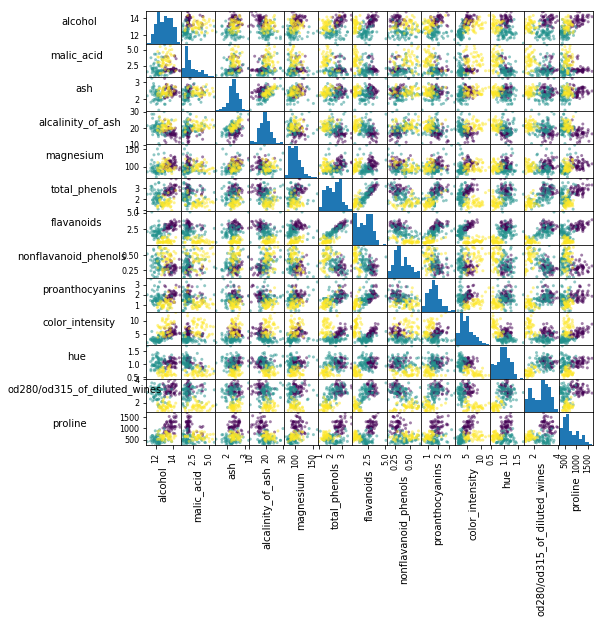#### Solution to Exercise 4

from sklearn.datasets import fetch_olivetti_faces

# fetch the faces data
faces = fetch_olivetti_faces()

faces.keys()

Output:
dict_keys(['data', 'images', 'target', 'DESCR'])
n_samples, n_features = faces.data.shape
print((n_samples, n_features))

(400, 4096)

np.sqrt(4096)

Output:
64.0
faces.images.shape

Output:
(400, 64, 64)
faces.data.shape

Output:
(400, 4096)
print(np.all(faces.images.reshape((400, 4096)) == faces.data))

True

# set up the figure
fig = plt.figure(figsize=(6, 6))  # figure size in inches
fig.subplots_adjust(left=0, right=1, bottom=0, top=1, hspace=0.05, wspace=0.05)

# plot the digits: each image is 8x8 pixels
for i in range(64):
ax = fig.add_subplot(8, 8, i + 1, xticks=[], yticks=[])
ax.imshow(faces.images[i], cmap=plt.cm.bone, interpolation='nearest')

# label the image with the target value
ax.text(0, 7, str(faces.target[i]))